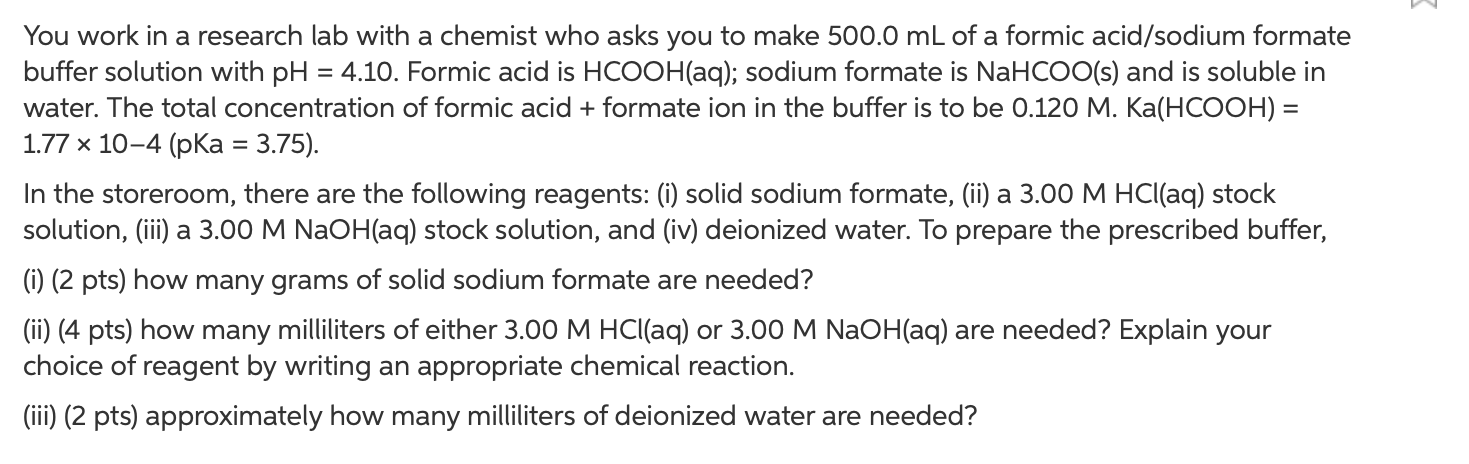# You work in a research lab with a chemist who asks you to make 500.0 mL of a formic acid/sodium formate buffer solution with pH = 4.10. Formic acid is HCOOH(aq); sodium formate is NaHCOO(s) and is soluble in water. The total concentration of formic acid + formate ion in the buffer is to be 0.120 M. Ka(HCOOH) = 1.77x10^-4 (pKa = 3.75). In the storeroom, there are the following reagents: (i) solid sodium formate, (ii)a 3.00 M HCl(aq) stock solution, (iii) a 3.00 M NaOH(aq) stock solution, and (iv) deionized water. To prepare the prescribed buffer, (i) how many grams of solid formate are needed? (ii) how many mL of either 3.00 M HCl(aq) or 3.00 M NaOH(aq) are needed? Explain your choice of reagent by writing an appropriate chemical reaction. (iii) approximately how many mL of deionized water are needed?